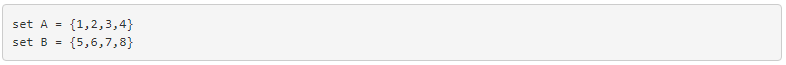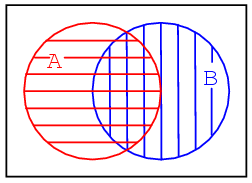# 8.2: Venn Diagrams and Sets

$$\newcommand{\vecs}{\overset { \rightharpoonup} {\mathbf{#1}} }$$ $$\newcommand{\vecd}{\overset{-\!-\!\rightharpoonup}{\vphantom{a}\smash {#1}}}$$$$\newcommand{\id}{\mathrm{id}}$$ $$\newcommand{\Span}{\mathrm{span}}$$ $$\newcommand{\kernel}{\mathrm{null}\,}$$ $$\newcommand{\range}{\mathrm{range}\,}$$ $$\newcommand{\RealPart}{\mathrm{Re}}$$ $$\newcommand{\ImaginaryPart}{\mathrm{Im}}$$ $$\newcommand{\Argument}{\mathrm{Arg}}$$ $$\newcommand{\norm}{\| #1 \|}$$ $$\newcommand{\inner}{\langle #1, #2 \rangle}$$ $$\newcommand{\Span}{\mathrm{span}}$$ $$\newcommand{\id}{\mathrm{id}}$$ $$\newcommand{\Span}{\mathrm{span}}$$ $$\newcommand{\kernel}{\mathrm{null}\,}$$ $$\newcommand{\range}{\mathrm{range}\,}$$ $$\newcommand{\RealPart}{\mathrm{Re}}$$ $$\newcommand{\ImaginaryPart}{\mathrm{Im}}$$ $$\newcommand{\Argument}{\mathrm{Arg}}$$ $$\newcommand{\norm}{\| #1 \|}$$ $$\newcommand{\inner}{\langle #1, #2 \rangle}$$ $$\newcommand{\Span}{\mathrm{span}}$$$$\newcommand{\AA}{\unicode[.8,0]{x212B}}$$

Mathematicians use Venn diagrams to show the logical relationships of sets (collections of objects) to one another. Perhaps you have already seen Venn diagrams in your algebra or other mathematics studies. If you have, you may remember overlapping circles and the union and intersection of sets. We will review the overlapping circles of the Venn diagram. We will adopt the terms OR and AND instead of union and intersection since that is the terminology used in digital electronics.

The Venn diagram bridges the Boolean algebra from a previous chapter to the Karnaugh Map. We will relate what you already know about Boolean algebra to Venn diagrams, then transition to Karnaugh maps.

A set is a collection of objects out of a universe as shown below. The members of the set are the objects contained within the set. The members of the set usually have something in common; though, this is not a requirement. Out of the universe of real numbers, for example, the set of all positive integers {1,2,3…} is a set. The set {3,4,5} is an example of a smaller set, or subset of the set of all positive integers. Another example is the set of all males out of the universe of college students. Can you think of some more examples of sets?Above left, we have a Venn diagram showing the set A in the circle within the universe U, the rectangular area. If everything inside the circle is A, then anything outside of the circle is not A. Thus, above center, we label the rectangular area outside of the circle A as A-not instead of U. We show B and B-not in a similar manner.

What happens if both A and B are contained within the same universe? We show four possibilities.Let’s take a closer look at each of the the four possibilities as shown above.The first example shows that set A and set B have nothing in common according to the Venn diagram. There is no overlap between the A and B circular hatched regions. For example, suppose that sets A and B contain the following members:None of the members of set A are contained within set B, nor are any of the members of B contained within A. Thus, there is no overlap of the circles.In the second example in the above Venn diagram, Set A is totally contained within set B How can we explain this situation? Suppose that sets A and B contain the following members:All members of set A are also members of set B. Therefore, set A is a subset of Set B. Since all members of set A are members of set B, set A is drawn fully within the boundary of set B.

There is a fifth case, not shown, with the four examples. Hint: it is similar to the last (fourth) example. Draw a Venn diagram for this fifth case.The third example above shows perfect overlap between set A and set B. It looks like both sets contain the same identical members. Suppose that sets A and B contain the following:Therefore,Sets And B are identically equal because they both have the same identical members. The A and B regions within the corresponding Venn diagram above overlap completely. If there is any doubt about what the above patterns represent, refer to any figure above or below to be sure of what the circular regions looked like before they were overlapped.The fourth example above shows that there is something in common between set A and set B in the overlapping region. For example, we arbitrarily select the following sets to illustrate our point:Set A and Set B both have the elements 3 and 4 in common These elements are the reason for the overlap in the center common to A and B. We need to take a closer look at this situation.

This page titled 8.2: Venn Diagrams and Sets is shared under a GNU Free Documentation License 1.3 license and was authored, remixed, and/or curated by Tony R. Kuphaldt (All About Circuits) via source content that was edited to the style and standards of the LibreTexts platform; a detailed edit history is available upon request.